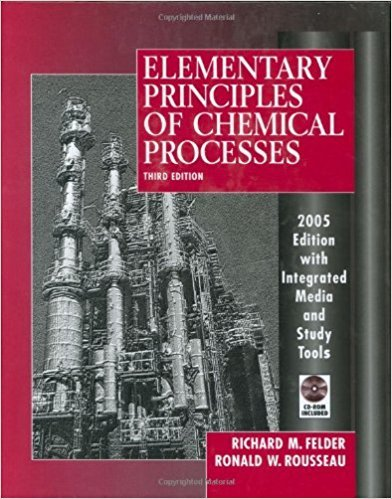×
Get Full Access to Elementary Principles Of Chemical Processes - 3 Edition - Chapter 3 - Problem 3.14
Get Full Access to Elementary Principles Of Chemical Processes - 3 Edition - Chapter 3 - Problem 3.14

×

# How many of the following are found in 15.0 kmol ofISBN: 9780471687573 143

## Solution for problem 3.14 Chapter 3

Elementary Principles of Chemical Processes | 3rd Edition

• Textbook Solutions
• 2901 Step-by-step solutions solved by professors and subject experts
• Get 24/7 help from StudySoup virtual teaching assistantsElementary Principles of Chemical Processes | 3rd Edition

4 5 1 269 Reviews
15
2
Problem 3.14

How many of the following are found in 15.0 kmol of benzene (C6H6 )? (a) kg C6H6 ; (b) mol C6H6 ; (c) lb-mole C6H6 ; (d) mol (g-atom) C; (e) mol H; (f) g C; (g) g H; (h) molecules of C6H6 .

Step-by-Step Solution:

Problem 3.14How many of the following are found in 15.0 kmol of benzene (C H ) (a) kg C 6 6 (b) mol 6 6C66 (c) lb-mole C66 (d) mol (g-atom) C; (e) mol H; (f) g C; (g) g H; (h) molecules of C H . 6 6 Step-by-step solution Step 1 of 8 ^(a)The moles of benzene (C H ) a665.0 kmol.The molecular weight of benzene is 78.114 kg/kmol. Multiply the moles of benzene with itsmolar mass to obtain mass of C H . 6 6 15.0 kmol C H6 6 78.114 kg C H 6 6 kmol C H = . 6 6

Step 2 of 8

Step 3 of 8

##### ISBN: 9780471687573

Unlock Textbook Solution